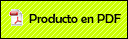A new computational model for the prediction of toxicity of phosphonate derivatives using QSPRAlvarado Rodríguez, José Guadalupe2018

A new computational model for the prediction of toxicity of phosphonate derivatives using QSPR. Camacho-Mendoza, R.L., Aquino-Torres, E., Cordero-Pensado, V., Cruz-Borbolla, J., Alvarado-Rodríguez, J.G., Thangarasu, P., Gómez-Castro, C.Z., Molecular Diversity, 12 (2018) 1-12

Abstract

Structural and electronic properties of a series of 25 phosphonate derivatives were analyzed applying density functional theory, with the exchange-correlation functional PBEPBE in combination with the 6-311++G** basis set for all atoms. The chemical reactivity of these derivatives has been interpreted using quantum descriptors such as frontier molecular orbitals (HOMO, LUMO), Hirshfeld charges, molecular electrostatic potential, and the dual descriptor [(Formula presented.)]. These descriptors are directly related to experimental median lethal dose ((Formula presented.), expressed as its decimal logarithm [(Formula presented.)((Formula presented.)] through a multiple linear regression equation. The proposed model predicts the toxicity of phosphonates in function of the volume (V), the load of the most electronegative atom of the molecule (q), and the eigenvalue of the molecular orbital HOMO ((Formula presented.). The obtained values in the internal validation of the model are: (Formula presented.)%, (Formula presented.)%, (Formula presented.), (Formula presented.), (Formula presented.), (Formula presented.), (Formula presented.), and (Formula presented.)%. The toxicity of nine phosphonate derivatives used as test molecules was adequately predicted by the model. The theoretical results indicate that the oxygen atom of the O=P group plays an important role in the interaction mechanism between the phosphonate and the acetylcholinesterase enzyme, inhibiting the removal of the proton of the ser-200 residue by the his-440 residue.Mild Oxidation of C?C Bonds of Benzoiridacycles

Complexation of the uranyl ion (UO2)2+ with a tetraphenylimidodiphosphinate ligand: the first trinuc...

Synthesis and crystal structure of the N-8-(diphenyl-hydroxy-2-aminomethylpyridine)borane

Synthesis, characterization, and crystal structures of n-alkyldiorganodithiophosphates RS2P(OC6H4)2

Study of the D?Sb (D = O, S) Transannular Interaction in Sb-Monohalogenated Dibenzostibocines ? An E...

Synthesis, characterization and crystal structures of dipyridyl-N-methylimine and cloro-bis (N,N?-2,...

Synthesis and crystal structures of cis-palladium(II) and cis-platinum(II) complexes containing dipy...

Synthesis and Relative Stability of a Series of Compounds of Type [Fe(II)(bztpen)X]+, Where bztpen =...

cis-Palladium(II) complexes of derivatives of di-(2-pyridyl)methane: Study of the influence of the b...

Ni(II) and Pd(II) organometallic and coordination complexes with a new tridentate N,N,O-donor ligand...